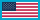Change Location# Electromagnetic Diffraction Modeling and Simulation with MATLAB

By (author)s: ,
Pages: 364
ISBN: 9781630817794

Hardback \$149.00 Qty:

This exciting new resource presents a comprehensive introduction to the fundamentals of diffraction of two-dimensional canonical structures, including wedge, strip, and triangular cylinder with different boundary conditions. Maxwell equations are discussed, along with wave equation and scattered, diffracted and fringe fields. Geometric optics, as well as the geometric theory of diffraction are explained. With MATLAB scripts included for several well-known electromagnetic diffraction problems, this book discusses diffraction fundamentals of two-dimensional structures with different boundary conditions and analytical numerical methods that are used to show diffraction.

The book introduces fundamental concepts of electromagnetic problems, identities, and definitions for diffraction modeling. Basic coordinate systems, boundary conditions, wave equation, and Green’s function problem are given. The scattered fields, diffracted fields, and fringe fields, radar cross section for diffraction modeling are presented. Behaviors of electromagnetic waves around the two-dimensional canonical wedge and canonical strip are also explored. Diffraction of trilateral cylinders and wedges with rounded edges is investigated as well as double tip diffraction using Finite Difference Time Domain and Method of Moments. A MATLAB based virtual tool, developed with graphical user interface (GUI), for the visualization of both fringe currents and fringe waves is included, using numerical FDTD and MoM algorithm and High-Frequency Asymptotics approaches.

Introduction; Two-Dimensional Canonical Wedge Problem; Two-Dimensional Canonical Strip Problem; Two-Dimensional Canonical Triangular Cylinder Problem; Diffraction at a Rectangular Plate; Diffraction with Rounded Edges; Double Tip Diffraction Modeling; WedgeGUI Virtual Package; FringeGUI Virtual Package; WedgeTOOL Virtual Package.

• Levent Sevgi Levent Sevgi is currently affiliated with the electrical and electronics engineering department of engineering and architecture at Okan University. He received his Ph.D. from the Weber Research institute at New York Polytechnic University York.
• Gökhan Apaydin

is professor at Uskudar University in Istanbul. He received his Ph.D from Bogazici University. He has written books and published many journal papers and is a senior member of IEEE.